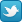# Linear Algebra Done Right

## Sheldon Axler

This best-selling textbook for a second course in linear algebra is aimed at undergraduate math majors and graduate students. The novel approach taken here banishes determinants to the end of the book. The text focuses on the central goal of linear algebra: understanding the structure of linear operators on finite-dimensional vector spaces. The author has taken unusual care to motivate concepts and to simplify proofs. A variety of interesting exercises in each chapter helps students understand and manipulate the objects of linear algebra.

No prerequisites are assumed other than the usual demand for suitable mathematical maturity. Thus the text starts by discussing vector spaces, linear independence, span, basis, and dimension. The book then deals with linear maps, eigenvalues, and eigenvectors. Inner product spaces are then introduced, leading to the finite-dimensional spectral theorem and its consequences such as the singular value decomposition. Generalized eigenvectors are then used to provide insight into the structure of a linear operator.

### Excerpts from Reviews

Altogether, the text is a didactic masterpiece.
zbMATH

Axler demotes determinants (usually quite a central technique in the finite dimensional setting, though marginal in infinite dimensions) to a minor role. To so consistently do without determinants constitutes a tour de force in the service of simplicity and clarity; these are also well served by the general precision of Axler's prose... The most original linear algebra book to appear in years, it certainly belongs in every undergraduate library.
Choice

The determinant-free proofs are elegant and intuitive.
American Mathematical Monthly

Clarity through examples is emphasized... the text is ideal for class exercises... I congratulate the author and the publisher for a well-produced textbook on linear algebra.
Mathematical Reviews

If you liked the previous editions, you will like this new edition even better!
Monatshefte für Mathematik• textbook adoptions: list of 341 universities and colleges that have adopted Linear Algebra Done Right as a textbookFollow @AxlerLinear on Twitter for updates on this book.

The print and Kindle versions of this book are available at amazon.com. Linear Algebra Done Right usually has the best Amazon sales rank of any linear algebra book at this level. The hardcover version of Linear Algebra Done Right is usually more than \$100 cheaper at Amazon than the hardcover version of the book by Friedberg/Insel/Spence, which usually has the second-best Amazon sales rank among linear algebra books at this level.

The print and eBook versions are also available at springer.com.

• If your library gets the appropriate Springer eBook package, then you can get the eBook version of this book for free at SpringerLink.

ISBN for print version: 978-3-319-11079-0

Linear Algebra Abridged is a free compactified version of Linear Algebra Done Right.
This book is partly based on ideas contained in my paper Down with Determinants!, published in the American Mathematical Monthly. This paper received the Lester R. Ford Award for expository writing from the Mathematical Association of America.
Faculty who are seriously interested in using this book as a text can contact me (`linear@axler.net`) to request that a review copy be sent from Springer.

Questions or comments about Linear Algebra Done Right can be sent to the author at `linear@axler.net`.

Sheldon Axler's publications